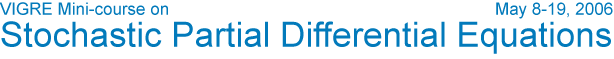Carl Mueller's Abstract Speakers Schedule & Notes Participants Visitor Information Contact Home Title: Qualitative properties of parabolic stochastic PDE Abstract: We will highlight some of the ideas used to study the qualitative behavior of parabolic stochastic PDE (SPDE). We will also mention related topics such as the wave equation. SPDE lies at the intersection of several different areas, and different groups have taken the subject in their own directions. For example, one could start with the functional analytic approach to PDE, and develop a similar framework for SPDE. Reasoning this way, one views solutions of SPDE as random variables taking values in Sobolev spaces (or other spaces), and the terms in the equations as operators and operator-valued random variables. Another possibility is to think of an SPDE as a stochastic process. In this approach, we focus on the intriguing properties of Brownian motion, martingales, the Brownian sheet, Gaussian processes, and other stochastic process that we know and love. I will call this point of view the Walsh approach, named after Walsh's St. Flour notes. To give a flavor of this approach, let us consider one particular process, the superprocess or Dawson-Watanabe process. Roughly speaking, this process solves the SPDE $\frac{\partial u}{\partial t} = \Delta u + \sqrt{u}\dot{W}(t,x).$ where $\dot{W}(t,x)$ is 2-parameter white noise. The superprocess arises as a limiting process in population dynamics and genetics. We will start with this connection, and see how it leads to an analysis of certain SPDE in terms of particles. Furthermore, ideas from particle systems, as given in Liggett's and Durrett's books, leads to useful information about SPDE. For example, certain SPDE exhibit phase transitions. One of the sources of SPDE was the Zakai equation of filtering theory, and there was some work on the stochastic Navier-Stokes equation, but the theory of SPDE itself was not closely tied to applications. Luckily, physicists and engineers have now caught up, and SPDE is appearing in more and more scientific fields. I may mention some of these connections, such as randomly growing surfaces in solid-state physics and the theory of randomly moving polymers. Also, the stochastic Navier-Stokes equation has received much more intensive study in recent years. Here is a possible list of further topics, in no particular order. I will cater to the interests of the audience. Connections to the Superprocess Information from the Integral Equation Large Deviations Duality Invariant Measures via Coupling and other Methods SPDE as limits of particle systems The Feynman-Kac Formula Similar Ideas for the Wave Equation and Other Equations Potential Theory Prerequisites: The first few chapters of Walsh's notes, or the equivalent. Some knowledge of superprocesses. VIGRE       U of U Math